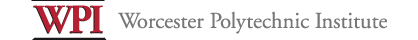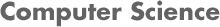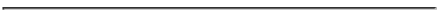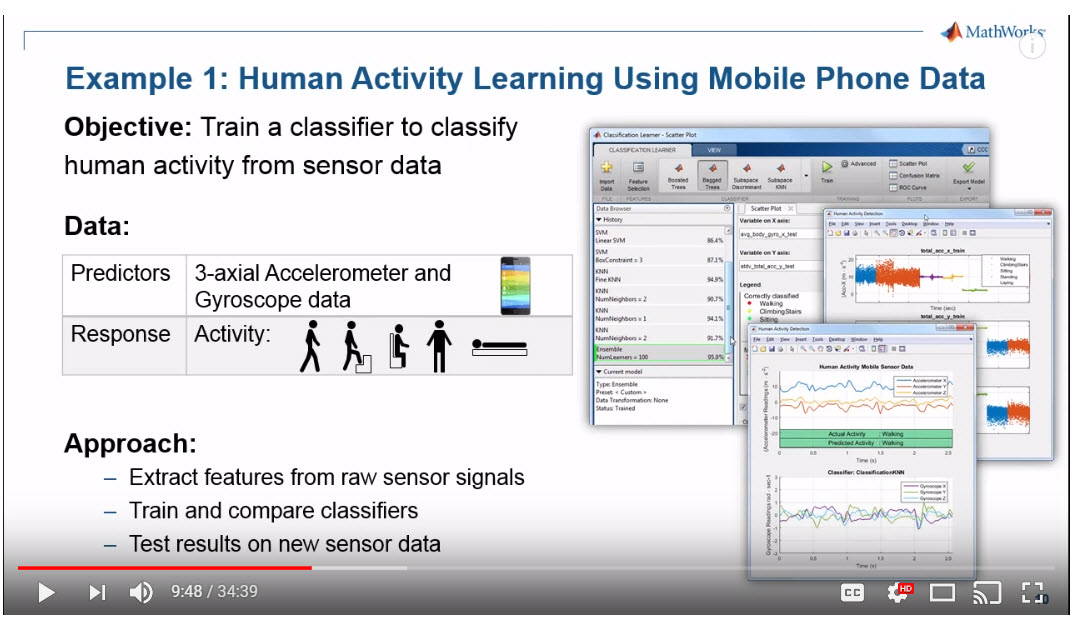## Overview

The aim of this project is to get you acquainted with machine learning classification of Human Activity using MATLAB. You should complete this project in your groups of 4 or 5 students. The GROUP will submit 1 project with all team members listed. You may discuss the projects with other classmates or on InstructAssist but each group will submit their own code for the project.

## Project Preparation

The following preparatory steps might be useful.

Step 1: Watch the Machine Learning Made Easy Video from MathWorks: This video provides an overview of how to do classification using the MATLAB machine learner app (a graphical user interface). MATLAB is installed on the zoolab machines. Click [ Here ] to go to the video. I created the following slides, which summarize the webinar. To access these slides, click [ Here ]Step 2: Download the code from the Webinar: MathWorks provided the code from the MATLAB webinar. The following zip file is the same zip file provided by MathWorks. Download it [ Here ]

Step 3: Run the Webinar Code and study it: Try to understand the code and all the steps. The code contains 2 examples:

• CarFinder: Detect which car type is in a given picture
• HumanActivity: Classification of human activity (Walking, Climbing stairs, Sitting, Standing, Laying down) from accelerometer and gyroscope data
For project 4, we are only interested in the Human Activity example.

## Project Requirements

Step 4: Implement new features: 3 features (Mean, Principal Component Analysis and Standard Deviation) are already implemented. You are required to implement additional features from the Activity Recognition Paper [ Activity Recognition using Cell Phone Accelerometers by Jennifer R. Kwapisz et al ] , which we previously studied. Specifically, implement the following features in separate MATLAB files:

• Average Absolute Difference
• Average Resultant Acceleration
• Time Between Peaks
• Binned Distribution

Separately, implement the features listed in table 2 of the paper [ A survey on human activity recognition using wearable sensors ] in separate MATLAB files. You will need to follow the paper's references for the exact formulas.

• Variance
• Interquartile range (IQR)
• Correlation between axes
• Entropy, and
• Kurtosis

Step 5: Answer the following questions: Create a README file and answer the following questions. Classify the activity using all classifier types that are available in the classification learner app. What is the 1) most accurate type of classifier and 2) Percentage accuracy when you use the following features:

1. only the 3 original features (mean, PCA and Standard deviation)?
2. 3 original features (mean, PCA, Standard deviation) and also Average Absolute Difference (i.e. 4 features in total)?
3. 3 original features (mean, PCA, Standard deviation) and also Average Absolute Difference and Average Resultant Acceleration (i.e. 5 features in total)?
4. 3 original features (mean, PCA, Standard deviation), and also Average Absolute Difference, Average Resultant Acceleration and Time Between Peaks (i.e. 6 features in total)?
5. 3 original features (mean, PCA, Standard deviation) and also Average Absolute Difference, Average Resultant Acceleration, Time Between Peaks and Binned Distribution (i.e. 7 features in total)?
6. 3 original features (mean, PCA, Standard deviation) and also Interquartilve Range (IQR) (i.e. 4 features in total)?
7. 3 original features (mean, PCA, Standard deviation) and Interquartile range (IQR), Mean Absolute Deviation (MAD), Correlation between axes, Entropy, and Kurtosis (i.e. 8 features in total)?
Using the holdout validation method, repeat question 5 above for test set percentage of 5%, 10%, 15%, 20%, 25% and 30%. What is the accuracy of the best performing classifier type? Which set of features were the most accurate (1-7)? Rank the feature sets in terms of their accuracy.